# Python | Pandas TimedeltaIndex.identical

Python is a great language for doing data analysis, primarily because of the fantastic ecosystem of data-centric python packages. Pandas is one of those packages and makes importing and analyzing data much easier.

Pandas` TimedeltaIndex.identical()` function determines if two Index objects contain the same elements. If the two objects under consideration contain same elements the function return True else the function return False.

Note : It is similar to equals, but check that other comparable attributes are also equal.

Syntax : TimedeltaIndex.identical(other)

Parameters :
other : other object

Return : boolean value

Example #1: Use `TimedeltaIndex.identical()` function to check if the elements contained in the two given TimedeltaIndex objects are identical or not.

 `# importing pandas as pd ` `import` `pandas as pd ` ` `  `# Create the first TimedeltaIndex object ` `tidx1 ``=` `pd.TimedeltaIndex(start ``=``'1 days 06:05:01.000030'``, periods ``=` `5``, ` `                                              ``freq ``=``'D'``, name ``=``'Koala'``) ` ` `  `# Create the second TimedeltaIndex object ` `tidx2 ``=` `pd.TimedeltaIndex(start ``=``'1 days 06:05:01.000030'``, periods ``=` `5``, ` `                                             ``freq ``=``'D'``, name ``=``'Koala'``) ` ` `  `# Print the first and second TimedeltaIndex object ` `print``(tidx1, ``'\n'``, tidx2) `

Output :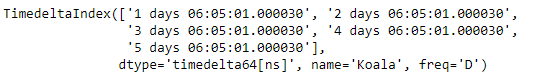Now we will use the `TimedeltaIndex.identical()` function to check if the values contained in tidx1 and tidx2 are identical or not.

 `# find if the elements are identical in tidx1 and tidx2 ` `tidx1.identical(tidx2) `

Output :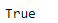As we can see in the output, the `TimedeltaIndex.identical()` function has returned `True` indicating that both the objects are identical to each other.

Example #2: Use `TimedeltaIndex.identical()` function to check if the elements contained in the two given TimedeltaIndex objects are identical or not.

 `# importing pandas as pd ` `import` `pandas as pd ` ` `  `# Create the TimedeltaIndex object ` `tidx1 ``=` `pd.TimedeltaIndex(data ``=``[``'1 days 02:00:00'``, ``'1 days 06:05:01.000030'``,  ` `                                 ``'1 days 02:00:00'``, ``'1 days 02:00:00'``, ` `                                           ``'21 days 06:15:01.000030'``]) ` ` `  `# Create the second TimedeltaIndex object ` `tidx2 ``=` `pd.TimedeltaIndex(data ``=``[``'06:05:01.000030'``, ``'+23:59:59.999999'``,  ` `                          ``'22 day 2 min 3us 10ns'``, ``'+12:19:59.999999'``]) ` ` `  `# Print the first and second TimedeltaIndex object ` `print``(tidx1, ``'\n'``, tidx2) `

Output :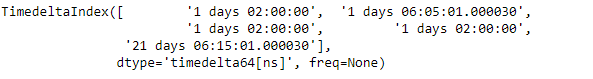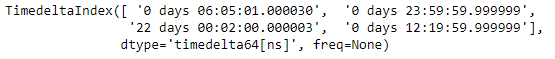Now we will use the `TimedeltaIndex.identical()` function to check if the values contained in tidx1 and tidx2 are same or not.

 `# find if the elements are identical in tidx1 and tidx2 ` `tidx.identical(tidx2) `

Output :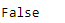As we can see in the output, the `TimedeltaIndex.identical()` function has returned `False` indicating that both the objects are not identical to each other.

My Personal Notes arrow_drop_upCheck out this Author's contributed articles.

If you like GeeksforGeeks and would like to contribute, you can also write an article using contribute.geeksforgeeks.org or mail your article to contribute@geeksforgeeks.org. See your article appearing on the GeeksforGeeks main page and help other Geeks.

Please Improve this article if you find anything incorrect by clicking on the "Improve Article" button below.

Article Tags :

Be the First to upvote.

Please write to us at contribute@geeksforgeeks.org to report any issue with the above content.